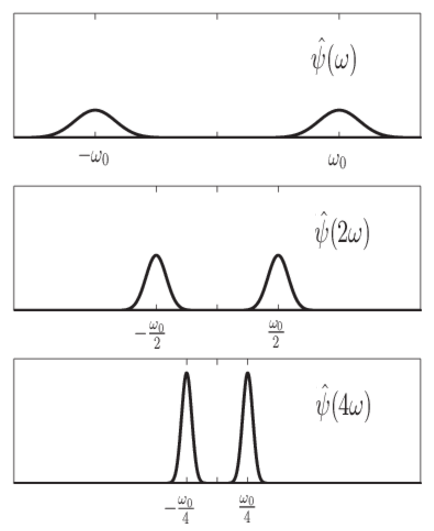## Continuous Wavelet Transform as a Bandpass Filter

### CWT as a Filtering Technique

The continuous wavelet transform (CWT) computes the inner product of a signal, $f\left(t\right)$, with translated and dilated versions of an analyzing wavelet, $\psi \left(t\right).$ The definition of the CWT is:

`$C\left(a,b;f\left(t\right),\psi \left(t\right)\right)={\int }_{-\infty }^{\infty }f\left(t\right)\frac{1}{a}{\psi }^{*}\left(\frac{t-b}{a}\right)dt$`

You can also interpret the CWT as a frequency-based filtering of the signal by rewriting the CWT as an inverse Fourier transform.

`$C\left(a,b;f\left(t\right),\psi \left(t\right)\right)=\frac{1}{2\pi }{\int }_{-\infty }^{\infty }\stackrel{^}{f}\left(\omega \right)\overline{\stackrel{^}{\psi }}\left(a\omega \right){e}^{i\omega b}d\omega$`

where $\stackrel{^}{f}\left(\omega \right)$ and $\stackrel{^}{\psi }\left(\omega \right)$ are the Fourier transforms of the signal and the wavelet.

From the preceding equations, you can see that stretching a wavelet in time causes its support in the frequency domain to shrink. In addition to shrinking the frequency support, the center frequency of the wavelet shifts toward lower frequencies. The following figure demonstrates this effect for a hypothetical wavelet and scale (dilation) factors of 1,2, and 4.This depicts the CWT as a bandpass filtering of the input signal. CWT coefficients at lower scales represent energy in the input signal at higher frequencies, while CWT coefficients at higher scales represent energy in the input signal at lower frequencies. However, unlike Fourier bandpass filtering, the width of the bandpass filter in the CWT is inversely proportional to scale. The width of the CWT filters decreases with increasing scale. This follows from the uncertainty relationships between the time and frequency support of a signal: the broader the support of a signal in time, the narrower its support in frequency. The converse relationship also holds.

In the wavelet transform, the scale, or dilation operation is defined to preserve energy. To preserve energy while shrinking the frequency support requires that the peak energy level increases. The implementation of `cwt` in Wavelet Toolbox™ uses L1 normalization. The quality factor, or Q factor of a filter is the ratio of its peak energy to bandwidth. Because shrinking or stretching the frequency support of a wavelet results in commensurate increases or decreases in its peak energy, wavelets are often referred to as constant-Q filters.

### DFT-Based Continuous Wavelet Transform

The equation in the preceding section defined the CWT as the inverse Fourier transform of a product of Fourier transforms.

`$C\left(a,b;f\left(t\right),\psi \left(t\right)\right)=\frac{1}{2\pi }{\int }_{-\infty }^{\infty }\stackrel{\wedge }{f}\left(\omega \right){\stackrel{^}{\psi }}^{*}\left(a\omega \right){e}^{j\omega b}d\omega$`

The time variable in the inverse Fourier transform is the translation parameter, b.

This suggests that you can compute the CWT with the inverse Fourier transform. Because there are efficient algorithms for the computation of the discrete Fourier transform and its inverse, you can often achieve considerable savings by using `fft` and `ifft` when possible.

To obtain a picture of the CWT in the Fourier domain, start with the definition of the wavelet transform:

`$=\frac{1}{a}{\int }_{-\infty }^{\infty }f\left(t\right){\psi }^{*}\left(\frac{t-b}{a}\right)dt$`

If you define:

`${\stackrel{˜}{\psi }}_{a}\left(t\right)=\frac{1}{a}{\psi }^{*}\left(-t/a\right)$`

you can rewrite the wavelet transform as

`$\left(f\ast {\stackrel{˜}{\psi }}_{a}\right)\left(b\right)={\int }_{-\infty }^{\infty }f\left(t\right){\stackrel{˜}{\psi }}_{a}\left(b-t\right)dt$`

which explicitly expresses the CWT as a convolution.

To implement the discretized version of the CWT, assume that the input sequence is a length N vector, x[n]. The discrete version of the preceding convolution is:

`${W}_{a}\left[b\right]=\sum _{n=0}^{N-1}x\left[n\right]\text{ }\text{ }{\stackrel{˜}{\psi }}_{a}\left[b-n\right]$`

To obtain the CWT, it appears you have to compute the convolution for each value of the shift parameter, b, and repeat this process for each scale, a.

However, if the two sequences are circularly-extended (periodized to length N), you can express the circular convolution as a product of discrete Fourier transforms. The CWT is the inverse Fourier transform of the product

`${W}_{a}\left(b\right)=\frac{1}{N}\sqrt{\frac{2\pi }{\Delta t}}\sum _{k=0}^{N-1}\stackrel{\wedge }{X}\left(2\pi k/N\Delta t\right)\stackrel{\wedge }{\psi }*\left(a2\pi k/N\Delta t\right){e}^{j2\pi kb/N}$`

where Δt is the sampling interval (period).

Expressing the CWT as an inverse Fourier transform enables you to use the computationally-efficient `fft` and `ifft` algorithms to reduce the cost of computing convolutions.

The `cwt` function implements the CWT.